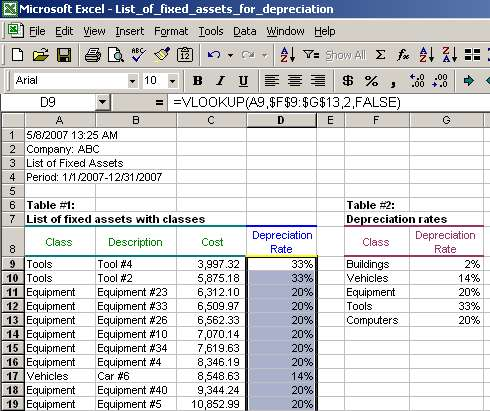# Vlookup Function, Vlookup Syntax, Vlookup Examples

Third, we need to ensure that our table_array does not shift when we copy the formula down. So, we need to change table_array cell references from relative to absolute by adding the dollar sign ("\$") before them as shown below (you can just type in those dollar signs):

=VLOOKUP(A9,\$F\$9:\$G\$13,2,FALSE)

In case we do not change the cell references from relative to absolute, the table_array will change when the VLOOKUP formula is copied from cell D9 to cells below.

Finally, we need to copy the formula for all fixed assets (till row 179). To do that, select cell D9, use key combination Ctrl+C to copy the formula, then select cells D10-D179 and use key combination Ctrl+V to paste the formula:The result is what we wanted - the list of fixed assets with depreciation rates assigned to each fixed asset. You can double check to see that any fixed asset with class Tools has depreciation rate of 33%, any fixed asset with class Equipment has depreciation rate of 20%, and so forth.

### 3) VLOOKUP applicability

The above example with depreciation rates is just one situation where VLOOKUP may serve you well and save you time. VLOOKUP may also be used in the following cases (which are by no means are all inclusive):

a) assigning cost to inventory quantities by using inventory master price list to calculate the inventory value (extended cost);
b) creating a comparative trial balance for a number of periods (refer to Comparative Trial Balances with Vlookup ); and
c) checking completeness of journal entries population by taking the beginning trial balance, adding debit and credit totals for all journal entries for a period and aligning the totals with the beginning trial balance, determining the estimated ending trial balance (beginning balance + debits - credits) and comparing it to the actual ending trial balance to see if the estimated and actual ending balances agree.

Page 3 of 3

Back